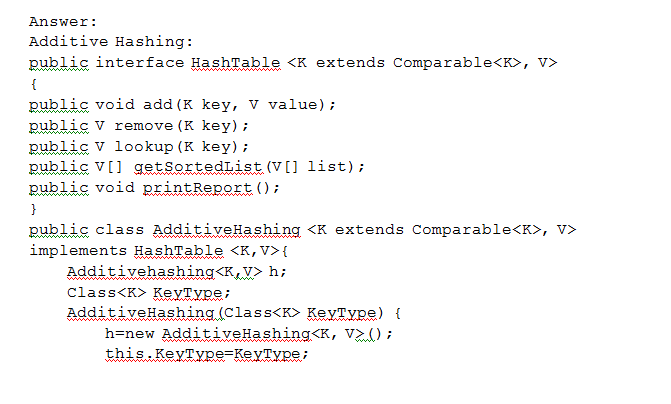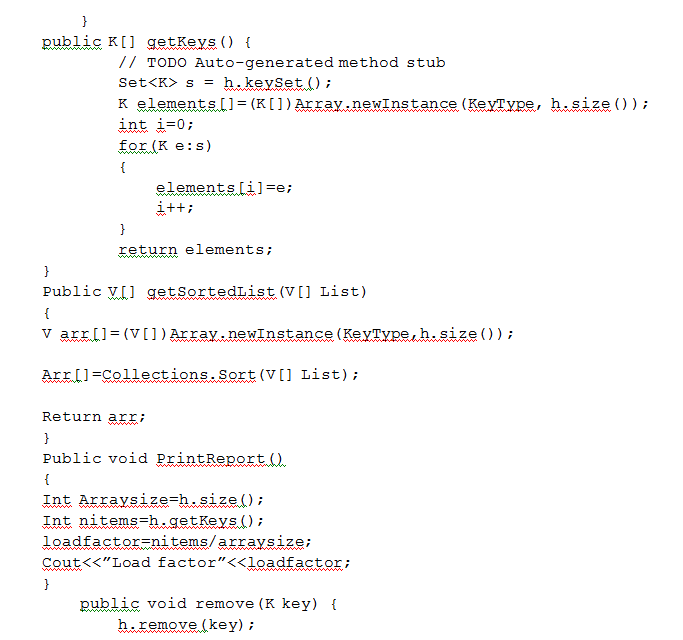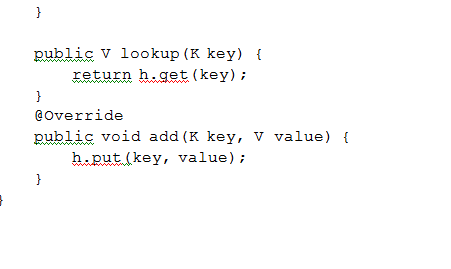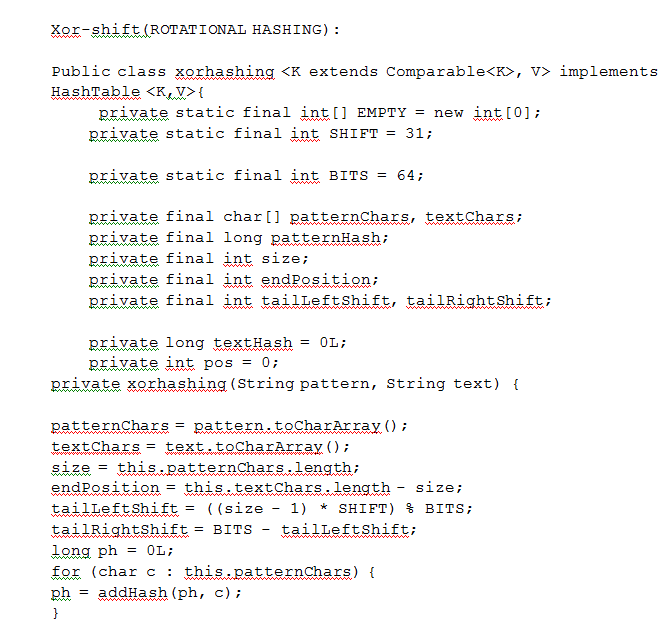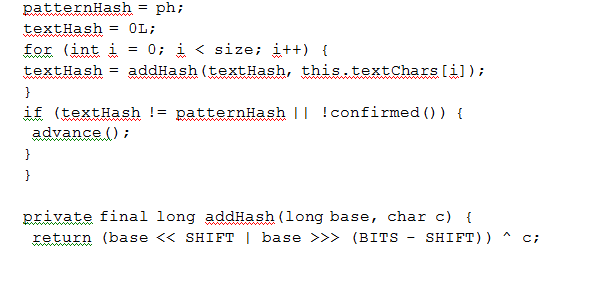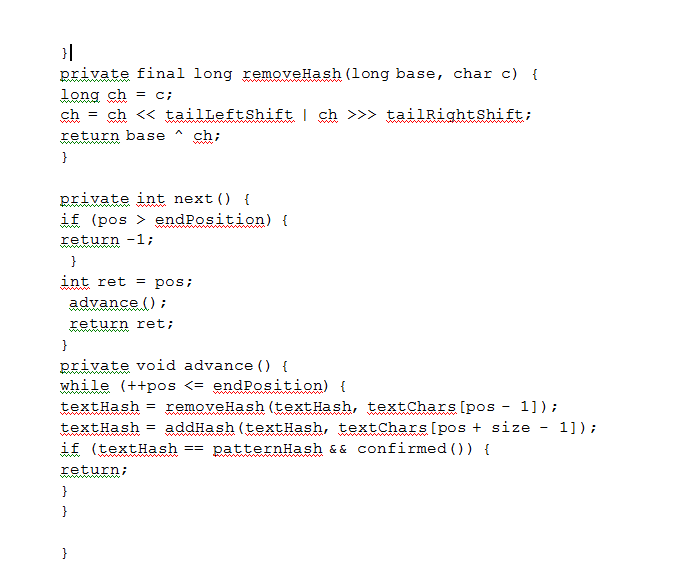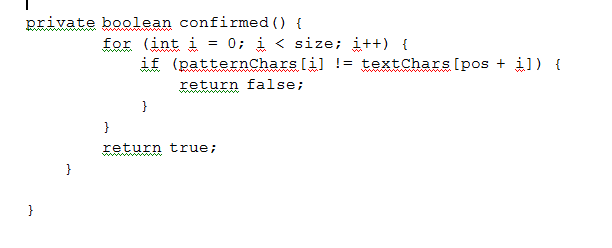# Answered! For HashTables, how do you implement both separate chaining and open addressing into this interface:…

For HashTables, how do you implement both separate chaining and open addressing into this interface:

public interface HashTable {

Don't use plagiarized sources. Get Your Custom Essay on
Answered! For HashTables, how do you implement both separate chaining and open addressing into this interface:…
GET AN ESSAY WRITTEN FOR YOU FROM AS LOW AS \$13/PAGE

public void add(String key, V value);

public V remove(String key);

public V lookup(String key);

public Object[] getValuesList();

public V[] getSortedList(V[] list);

public void printReport();

}

The printReport() method should print to the console the following statistics:
– The Load Fator, that is, the ratio of used to total number of buckets.
– The longest chain in the table, that is, the maximum number of collisions for a particular bucket.
– The Density Factor, that is, the ratio of elements stored elements to total number of buckets.
– The Chanining Factor, that is, the average length of any chain in the table.

Here is the code for the hash functions that are to be implemented:

public int additiveHash(char[] key, int TABLE_SIZE) {
int hash = 0;

for (char c: key) {
hash += c;
}

return hash % TABLE_SIZE;

}

public int rotationalHash(char[] key, int TABLE_SIZE) {
int hash = 0;

for (char c: key) {
hash += (c << 7) ^ (c >> (TABLE_SIZE – 7)) ^ hash;
}

hash = Math.abs(hash);

return hash % TABLE_SIZE;
}